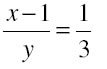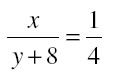# Form the pair of linear equations in the following problems and find their solutions (if they exist) by any algebraic method :

1.5k views

(i) A part of monthly hostel charges is fixed and the remaining depends on the number of days one has taken food in the mess. When a student A takes food for 20 days she has to pay Rs 1000 as hostel charges whereas a student B, who takes food for 26 days, pays Rs 1180 as hostel charges. Find the fixed charges and the cost of food per day.

(ii) A fraction becomes 1/3 when 1 is subtracted from the numerator and it becomes 1/4 when 8 is added to its denominator. Find the fraction.

by (48 points)

Let fixed charge = Rs x
Variable charge  = Rs y per day
When a student Atakes food for 20 days she has to pay Rs 1000 as hostel charges
So we get
X  + 20 y = 1000       …(1)
Subtract 20 y both side we get
X = 1000 – 20 y        …(2)
who takes food for 26 days, paysRs 1180 as hostel charges
we get
x + 26 y = 1180
plug the value of x we ge t

1000 – 20 y + 26y = 1180
6y = 180
Y = 180/6 = 30

Plug the value of y in equation second we get
X = 1000 – 20 * 30
X = 1000 – 600
X = 400
Hence fixed charge x  = Rs 400
And variable charge y = Rs 30

2 Let fraction is x/y
A fraction becomes 1/3 when 1 is subtracted from the numerator
So thatCross multiply we get
3x -3  = y                    …(1)
and it becomes 1/ 4 when 8 is added to its denominator
so that4x = y + 8
Plug the value of y from equation first we get
4x = 3x – 3 + 8
X = 5
Plug this value back in equation first we get
3*5 – 3  = y
15 – 3 = y
12 = y
Hence fraction is x/y = 5/12